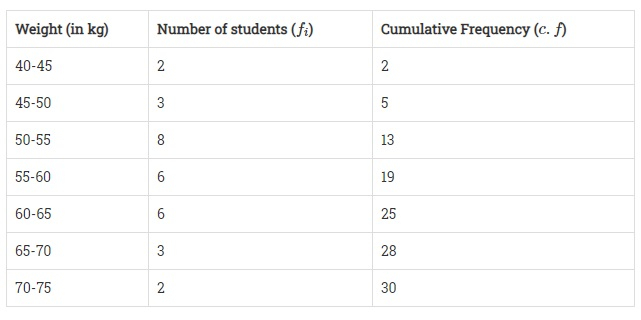Weight (in kg):40-4545-5050-5555-6060-6565-7070-75No. of students:2386632"">

# The distribution below gives the weight of 30 students of a class. Find the median weight of the students.Weight (in kg):40-4545-5050-5555-6060-6565-7070-75No. of students:2386632"

Given:

The distribution gives the weight of 30 students in a class.

To do:

We have to find the median weight of students.

Solution:Here,

$N = 30$

$\frac{N}{2} = \frac{30}{2} = 15$

The cumulative frequency just greater than $\frac{N}{2}$ is 19 and the corresponding class is 55 – 60.

This implies, that 55– 60 is the median class.

Therefore,

$l = 55, f = 6, F = 13$ and $h = (60 - 55) = 5$

Median $=\mathrm{l}+\frac{\frac{\mathrm{N}}{2}-\mathrm{F}}{\mathrm{f}} \times \mathrm{h}$

$=55+\frac{15-13}{6} \times 5$

$=55+\frac{2}{6} \times 5$

$=55+\frac{5}{3}$

$= 55 + 1.67$

$= 56.67$

The median weight of students is 56.67 kg.

Updated on: 10-Oct-2022

88 Views